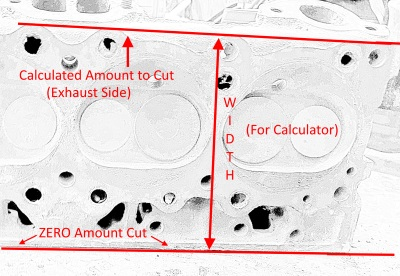Calculate Amount to Angle Mill Head Per Degree

 This calculator will calculate the amount that needs to be milled for a different angle on the head deck. The angle is the angle you want to cut off, say 1º on a 23º Chevy head. You would enter 1 into the Angle input. The width is the surface that will be cut from the intake side to the exhaust side of the head. (The widest part that would be milled) The amount calculated would be the amount to cut from the exhaust side of the head going to zero amount being cut on the intake side. (which would give the degree which you want of the angle cut) One aspect you need to remember is that the intake face of the head will also change by the degree you mill the head deck surface. (if you angle mill 1º, the intake face will change 1º also at the intake face) Using this calculator could be used to figure that amount also. Use the angle inputted previously along with the width of the intake side of the head surface. Starting from zero on bottom side of head/intake to the cut amount computed to the top of the head/intake.Calculate Amount to Angle Mill Head Enter Angle To Mill: Degrees Enter Width of Head Deck: Inches Input All Data, Please